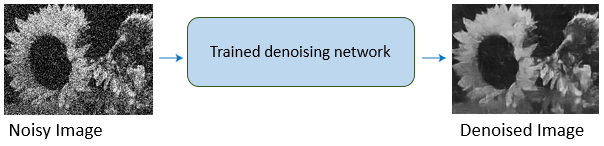# Deep Learning for Image Processing

Perform image processing tasks, such as removing image noise and performing image-to-image translation, using deep neural networks (requires Deep Learning Toolbox™)

Deep learning uses neural networks to learn useful representations of features directly from data. For example, you can use a pretrained neural network to identify and remove artifacts like noise from images.## Functions

expand all

 `augmentedImageDatastore` Transform batches to augment image data `blockedImageDatastore` Datastore for use with blocks from `blockedImage` objects (Since R2021a) `denoisingImageDatastore` Denoising image datastore `imageDatastore` Datastore for image data `randomPatchExtractionDatastore` Datastore for extracting random 2-D or 3-D random patches from images or pixel label images `transform` Transform datastore (Since R2019a) `combine` Combine data from multiple datastores (Since R2019a)
 `jitterColorHSV` Randomly alter color of pixels (Since R2019b) `randomWindow2d` Randomly select rectangular region in image (Since R2021a) `randomCropWindow3d` Create randomized cuboidal cropping window (Since R2019b) `centerCropWindow2d` Create rectangular center cropping window (Since R2019b) `centerCropWindow3d` Create cuboidal center cropping window (Since R2019b) `Rectangle` Spatial extents of 2-D rectangular region (Since R2019b) `Cuboid` Spatial extents of 3-D cuboidal region (Since R2019b) `randomAffine2d` Create randomized 2-D affine transformation (Since R2019b) `randomAffine3d` Create randomized 3-D affine transformation (Since R2019b) `affineOutputView` Create output view for warping images (Since R2019b) `imerase` Remove image pixels within rectangular region of interest (Since R2021a)
 `resize2dLayer` 2-D resize layer (Since R2020b) `resize3dLayer` 3-D resize layer (Since R2020b) `dlresize` Resize spatial dimensions of `dlarray` object (Since R2020b) `DepthToSpace2DLayer` Depth to space layer (Since R2021a) `SpaceToDepthLayer` Space to depth layer (Since R2020b) `depthToSpace` Rearrange `dlarray` data from depth dimension into spatial blocks (Since R2021a) `spaceToDepth` Rearrange spatial blocks of `dlarray` data along depth dimension (Since R2021a)
 `encoderDecoderNetwork` Create encoder-decoder network (Since R2021a) `blockedNetwork` Create network with repeating block structure (Since R2021a) `pretrainedEncoderNetwork` Create encoder network from pretrained network (Since R2021a) `cycleGANGenerator` Create CycleGAN generator network for image-to-image translation (Since R2021a) `patchGANDiscriminator` Create PatchGAN discriminator network (Since R2021a) `pix2pixHDGlobalGenerator` Create pix2pixHD global generator network (Since R2021a) `addPix2PixHDLocalEnhancer` Add local enhancer network to pix2pixHD generator network (Since R2021a) `unitGenerator` Create unsupervised image-to-image translation (UNIT) generator network (Since R2021a) `unitPredict` Perform inference using unsupervised image-to-image translation (UNIT) network (Since R2021a)
 `denoiseImage` Denoise image using deep neural network `denoisingNetwork` Get image denoising network `dnCNNLayers` Get denoising convolutional neural network layers

## Topics

### Deep Learning in MATLAB

• Deep Learning in MATLAB (Deep Learning Toolbox)
Discover deep learning capabilities in MATLAB using convolutional neural networks for classification and regression, including pretrained networks and transfer learning, and training on GPUs, CPUs, clusters, and clouds.
• Semantic Segmentation Using Deep Learning (Computer Vision Toolbox)
This example shows how to segment an image using a semantic segmentation network.